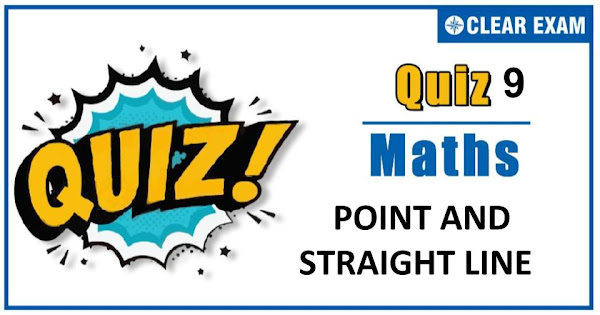## [LATEST]\$type=sticky\$show=home\$rm=0\$va=0\$count=4\$va=0

Dear Readers, As per analysis for previous years, it has been observed that students preparing for JEE MAINS find Mathematics out of all the sections to be complex to handle and the majority of them are not able to comprehend the reason behind it. This problem arises especially because these aspirants appearing for the examination are more inclined to have a keen interest in Mathematics due to their ENGINEERING background.
Furthermore, sections such as Mathematics are dominantly based on theories, laws, numerical in comparison to a section of Engineering which is more of fact-based, Physics, and includes substantial explanations. By using the table given below, you easily and directly access to the topics and respective links of MCQs. Moreover, to make learning smooth and efficient, all the questions come with their supportive solutions to make utilization of time even more productive. Students will be covered for all their studies as the topics are available from basics to even the most advanced.
.

Q1. L is variable line such that the algebraic sum of the distances of the points (1, 1), (2, 0) and (0,2) from the line is equal to zero. The line L will always pass through
•  (1, 1)
•  (2, 1)
•  (1,2)
•  (2, 2)
Solution
(a) Let the equation of line be ax+by+c=0 …(i) The perpendicular distance from (1, 1), (2, 0) and (0, 2) to the line ax+by+c=0 are p_1=(a+b+c)/√(a2+b2 ),p_2=(2a+c)/√(a2+b2,/sup>),p3=(2b+c)/√(a2+b2 ) Since, it is given that p1+p2+p3=0 ⇒(a+b+c)/√(a2+b2)+(2a+c)/√(a2+b2)+(2b+c)/(a2+b2)=0 ⇒3a+3b+3c=0 ⇒a+b+c …(ii) From Eq. (ii), it is clear that the line (i) passes through (1, 1)
Q2.If one of the lines of my2+(1-m2)xy- mx2=0 is a bisector of the angle between the lines xy=0, then m is/are
•  -1/2
•  -2
•  ±1
•   2
Solution
c) Equation of bisectors of lines xy=0 are y=±x. Put y=±x in my2+(1-m2)xy-mx2=0, we get mx2±(1-m2)x2 - mx2=0 ⇒ (1-m2 )x2=0 ⇒ m=±1
Q3.  The distance between the lines 3x+4y=9 and 6x+8y=15 is
•  3/2
•  3/10
•  6
•  None of these
Solution

b) Given lines are 3x+4y=9 …(i) and 6x+8y=15 ⇒3x+4y=15/2 …(ii) ∵ Both lines are parallel, therefore the distance between two lines=|15/2-9|/√(3^2+4^2 )=3/(2∙5)=3/10

Q4. A straight rod of length 9 units slides with its ends A,B always on the X and Y axis respectively. then, the locus of the centroid of ∆ OAB is
•  x2+y2=3
•  x2+y2=9
•  x2+y2=1
•  x2+y2=81
Solution
b) Let P(h,k) be the centroid of ∆ OAB. Let the coordinates of A and B be (a,0) and (0,b) respectively. then, h=a/3,k=b/3 in ∆ OAB, we have OA2+OB2=AB2 ⇒a2+b2=92⇒9h2+9k2=92⇒h2+k2=9 Hence, the locus of (h,k) is x2+y2=9
Q5.The angle between the straight line x-y√3=5 and √3 x+y=7 is
•  90°
•  60°
•  75°
•  30°
Solution
(a) Given equation is compared with a1 x+b1 y=0 and a2 x+b2 y=0 Now, a1a2+b1b2=(1)(√3)+(-√3)(1)=0 ∴ Lines are perpendicular Hence, θ=90°
Q6.  The length of perpendicular from the point (a cos⁡α,a sin⁡α) upon the straight line y=x tan⁡α+c,c>0, is
•  c
•   c sin2α
•  c cos⁡α
•  c sec2α
Solution
(c) The given line is x tan⁡α-y+c=0 or x sin⁡α-y cos⁡α+c cos⁡α=0 ∴ Length of perpendicular from (a cos⁡α,a sin⁡α ) =(a cos⁡α sin⁡α-a sin⁡α cos⁡α+c cos⁡α)/√(sin 2 α+cos2 α) =(c cos⁡α)/1=c cos⁡α
Q7.If the foot of the perpendicular from the origin to a straight line is at the point(3,-4). Then, the equation of the line is
•  3x-4y=25
•  3x-4y+25=0
•  4x+3y-25=0
•  4x-3y+25=0
Solution
a) Let P(3,-4) be the foot of the perpendicular from the origin O on the required line. Then,the slope of OP=(-4-0)/(3-0)=(-4)/3 Therefore, the slope of the required line is 3/4 Hence,its equation is y+4=3/4 (x-3) ⇒ 3x-4y-9-16=0 ⇒ 3x-4y=25
Q8.Locus of the centroid of triangle whose vertices are (a cos⁡t,a sin⁡t),(b sin⁡t,-b cos⁡t) and (1,0), where t is a parameter, is
•  (3x-1)2+(3y)2=a2-b2
•  (3x-1)2+(3y)2=a2+b2
•  (3x+1)2+(3y)2=a2+b2
•  (3x+1)2+(3y)2=a2-b2
Solution

(b) Let (h,k) be the centroid of the triangle whose vertices are (a cos⁡t,a sin⁡t),(b sin⁡t,-b cos⁡t ) and (1,0). Then, 3h=a cos⁡t+b sin⁡t+1 and 3k=a sin⁡t-b cos⁡t ⇒(3h-1)2+(3k)2=a2+b2 Hence, the locus of (h,k) is (3x-1)2+(3y)2=a2+b2
Q9.  Area of the parallelogram formed by the lines y=mx,y=mx+1,y=nx and y=nx+1 equals
•  (|m+n|)/(m-n)2
•  2/(|m+n|)
•  1/|m+n|
•  1/(|m-n|)
Solution
(d)
Q10. The line 2x-y=1 bisects angle between two lines. If equation of one line is y=x, then the equation of the other line is
•   7x-y-6=0
•   x-2y+1=0
•  3x-2y-1=0
•  x-7y+6=0
Solution
(b) Let the another equation of line is x-2y+1=0 ∴ Equation of bisector of angle between two lines is (2x-y-1)/√(4+1)=±(x-2y+1)/√(1+4)#### Written by: AUTHORNAME

AUTHORDESCRIPTION## Want to know more

Please fill in the details below:

## Latest NEET Articles\$type=three\$c=3\$author=hide\$comment=hide\$rm=hide\$date=hide\$snippet=hide

Name

ltr
item
BEST NEET COACHING CENTER | BEST IIT JEE COACHING INSTITUTE | BEST NEET, IIT JEE COACHING INSTITUTE: Point and Straight Line Quiz-9
Point and Straight Line Quiz-9
https://1.bp.blogspot.com/-XF75Jh8V4Aw/X6VAPNpRYAI/AAAAAAAAKcY/VBvFmp4RpdoOiYuaubS0Vs0j3QOrafgqQCLcBGAsYHQ/s600/Quiz%2BImage%2B20%2B%252810%2529.jpg
https://1.bp.blogspot.com/-XF75Jh8V4Aw/X6VAPNpRYAI/AAAAAAAAKcY/VBvFmp4RpdoOiYuaubS0Vs0j3QOrafgqQCLcBGAsYHQ/s72-c/Quiz%2BImage%2B20%2B%252810%2529.jpg
BEST NEET COACHING CENTER | BEST IIT JEE COACHING INSTITUTE | BEST NEET, IIT JEE COACHING INSTITUTE
https://www.cleariitmedical.com/2020/11/point-and-straight-line-quiz-9.html
https://www.cleariitmedical.com/
https://www.cleariitmedical.com/
https://www.cleariitmedical.com/2020/11/point-and-straight-line-quiz-9.html
true
7783647550433378923
UTF-8